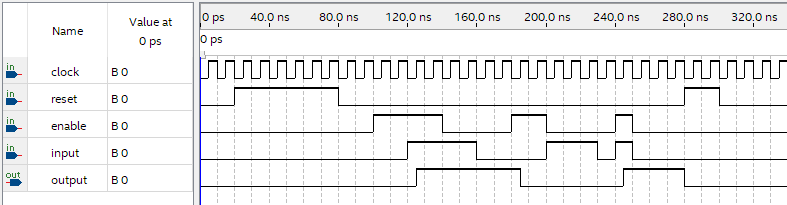# Preamble

VHDL stands for Very High Speed Integrated Circuit Hardware Design Language. No, I’m not kidding. Despite being a mouthful, the language is used by engineers to prototype and describe industrial digital logic.

This document is a copy of living notes for the CEG3155 course I am currently taking, Digital Systems II.

The two primary types of VHDL logic the course deals with (and I deal with within this document) are called concurrent and sequential, where concurrent logic obviously runs concurrently, and sequential logic runs sequentially within process statements.

# A Basic VHDL Program

Let’s dive right in with a full example. Here is an implementation for a decoder with an enable bit. Note the two primary sections of the program: entity, and architecture.

``````library ieee;
use ieee.std_logic_1164.all;

entity decoder_enable is
port(
a, b, en : in std_logic;
d : out std_logic_vector(3 downto 0)
);
end decoder_enable;

architecture de_arch of decoder_enable is
begin
d <= "0000" when (en="0") else
"0001" when (not(a or b)) else
"0010" when ((not a) and b) else
"0100" when (a and (not b)) else
"1000" when (a and b);
end de_arch;``````

The first thing that our program requires is the standard IEEE 1164 libraries. These should always be present at the top of your programs to use constructs like `std_logic`.

``````library ieee;
use ieee.std_logic_1164.all;``````

After importing libraries, we describe our entity by providing the inputs and outputs. Here, `a`, `b`, and `en` are of type `std_logic`, a simple binary operator that can hold `0` or `1`. Our output is a `std_logic_vector`, which is an array of `std_logic` objects. The notation `3 downto 0` will create four output bits.

``````entity decoder_enable is
port(
a, b, en : in std_logic;
d : out std_logic_vector(3 downto 0)
);
end decoder_enable;``````

After describing our entity, we describe the internals of the entity with an architecture block. The output `d` is written to with the arrow operator `<=`, and using `when` and `else` statements. The first condition that is true will be written to the output `d`.

``````architecture de_arch of decoder_enable is
begin
d <= "0000" when (en="0") else
"0001" when (not(a or b)) else
"0010" when ((not a) and b) else
"0100" when (a and (not b)) else
"1000" when (a and b);
end de_arch;``````

This logic is technically concurrent. Each assignment (`<=`) will run simultaneously when the signal reaches the component. Note that only a single entity can be defined per VHDL file.

# Internal Signals

Signals can be used to redirect logic within a component. Inputs cannot be written to, and outputs cannot be used for input to other systems, so signals are used to ferry internal outputs to internal inputs.

``````--- Assume w, x, y, z are used as inputs.
architecture arch of and_4 is
signal a, b : std_logic;

begin
a <= w and x;
b <= y and z;
output <= a and b;
end arch;``````

# Inclusion of Components

Large digital systems can be implemented by combining large amounts of components defined in other VHDL files. We accomplish this with internal signals and component statements.

For instance, a trivial NAND component with one input and output can be defined in its own VHDL file, like so:

``````library ieee;
use ieee.std_logic_1164.all;

entity nand is
port(
x, y : in std_logic;
z : out std_logic
);
end decoder_enable;

architecture nand_arch of nand is
begin
y <= not (x and y);
end nand_arch;``````

If you were to use this in another VHDL architecture, you would first have to place `nand.vhdl` in the same directory, then you could write an architecture like the following:

``````architecture sample_arch of big_component is
signal H : std_logic_vector(1 downto 0);

component NAND
port(
x, y : in std_logic;
z : out std_logic
);
end component;

begin
L0: NAND(SW(0), SW(1), H);
LEDR(0) <= H;
end sample_arch;``````

# Concurrent/Structural VHDL

Concurrent statements are used to model real systems. For instance, a D Flip Flop can be modeled in structural style:

``````library ieee;
use ieee.std_logic_1164.all;

entity register_1 is
port(
input, enable, clock, reset : in std_logic;
output : out std_logic
);
end register_1;

architecture structural of register_1 is
begin

output <= '0' when (reset = '1') else
input when ( rising_edge(clock) and enable = '1');

end structural;``````For an architecture to be considered as structural, process statements cannot be used within the body of the primary architecture.

# Sequential/Behavioural VHDL

Process statements can be used to define logic with a familiar, imperative style. It is fine for creating test benches, but should not be used for modeling components which will be made into hardware.